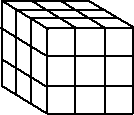# Maths - Hypermatrix

Since vectors can be thought of as a 1 x n array of elements, and a matrix is an n x m array of elements, then why not continue this sequence to mathematical objects with n x m x p elements and n x m x p ...What would be the multiplication rules for such an algebra?

I can't think of an application for this (unless it is the same as a tensor?) in which case it is used extensively in electromagnetic fields, relativity, quantum mechanics and particle physics.

Could it be, for instance, by analogy with a linear equation, where a matrix transforms on vector into another:

 Vout 0 Vout 1 Vout 2 Vout 3
=
 m00 m01 m02 m03 m10 m11 m12 m13 m20 m21 m22 m23 m30 m31 m32 m33
 Vin 0 Vin 1 Vin 2 Vin 3

that a hypermatrix might transform one matrix into another (multi-linear algebra?):

 mo00 mo01 mo02 mo10 mo11 mo12 mo20 mo21 mo22
=mi00 mi01 mi02 mi10 mi11 mi12 mi20 mi21 mi22

Of course we can transform a 3x3 matrix into another 3x3 matrix by multiplying it by a third 3x3 matrix. Would it be more general to multiply it by a hypermatrix?

Or is it possible to have a matrix equivalent of multivectors (Clifford algebra) with two types of multiplication. An inner product which transforms:

real number <-- vector <--- matrix <-- hypermatrix

and an outer product which transforms:

real number --> vector ---> matrix --> hypermatrix

## What is the relationship between a hypermatrix and a tensor?

Is a hypermatrix just a representation of a tensor?

I think that perhaps a tensor must be square, cuboid, ... In other words it must be the same size in each dimention?

So perhaps not all hypermatrices are tensors?

Rank representation element notation
0 scalar a
1 vector ai
2 matrix aij
3 hypermatrix of rank 3 aijk

 metadata block see also: Correspondence about this page Book Shop - Further reading. Where I can, I have put links to Amazon for books that are relevant to the subject, click on the appropriate country flag to get more details of the book or to buy it from them.Schaum's Outline of Theory and Problems of Tensor Calculus - I'm finding this hard going, it starts off with as review of linear algebra, matrix notation, etc. It redefines a lot of conventions which are hard to relearn, such as superscrips instead of subscripts to identify elements, and a summation convention, then it goes into coordinate transformations. I cant find any definition of what a tensor is. I think this book is aimed at people who already have some knowledge of the subject. I can't find any mention in this book of the term hyper-matrix. Specific to this page here:

This site may have errors. Don't use for critical systems.